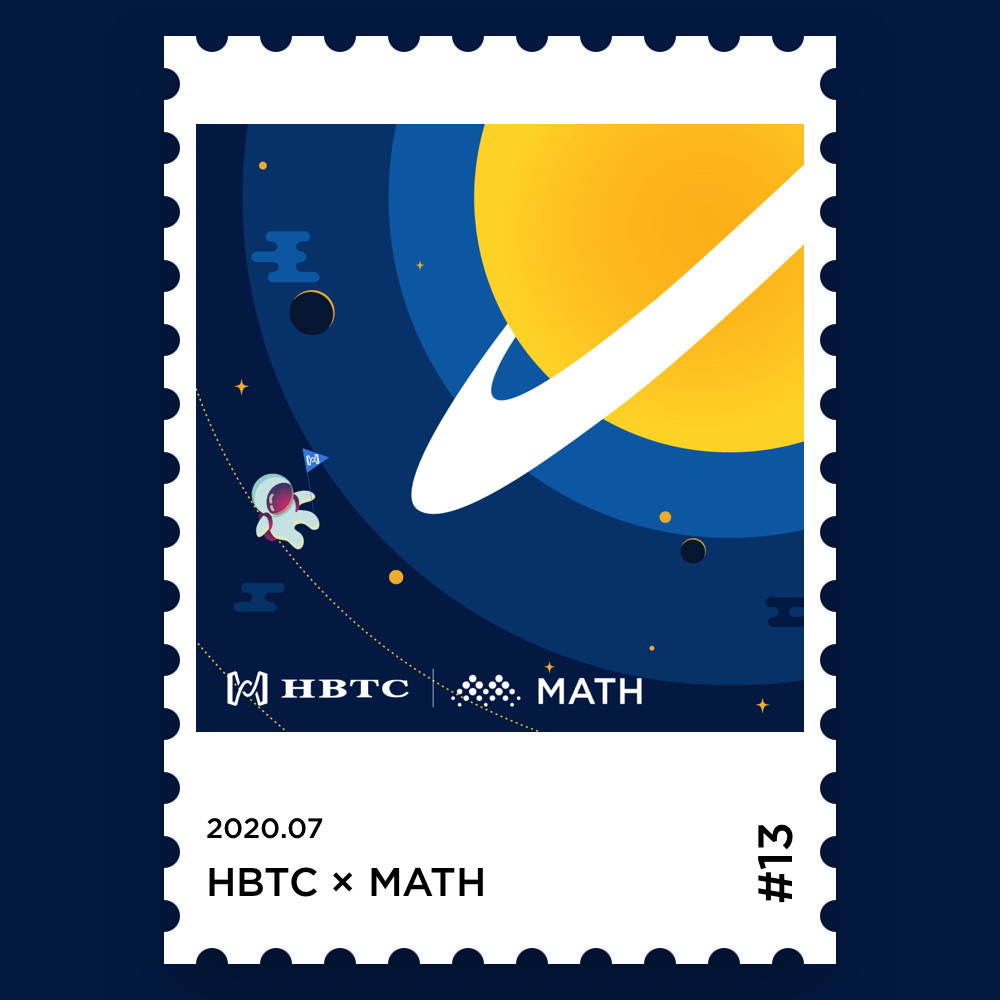# MATH NFT Collection

### 001 MATH x ETHEREUM002 MATH x CHAINNEWS003 MATH x MXC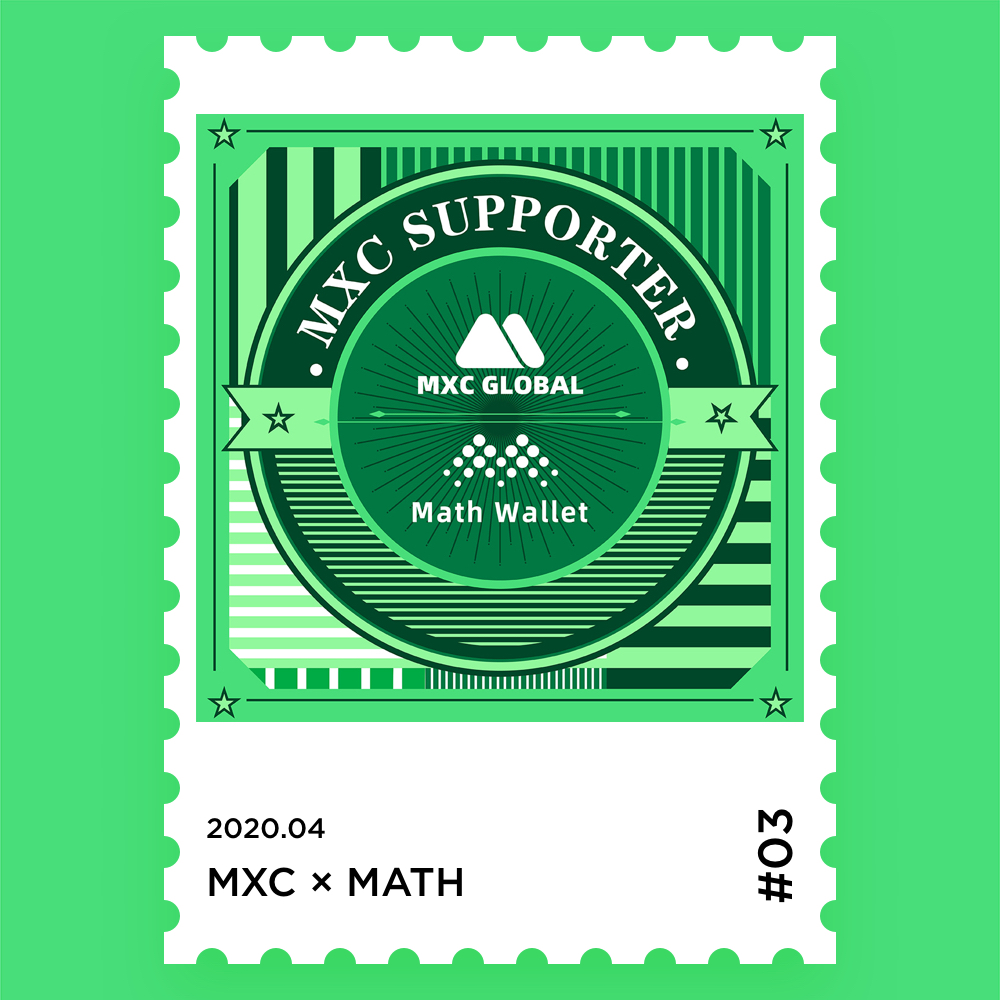004 MATH x GXCHAIN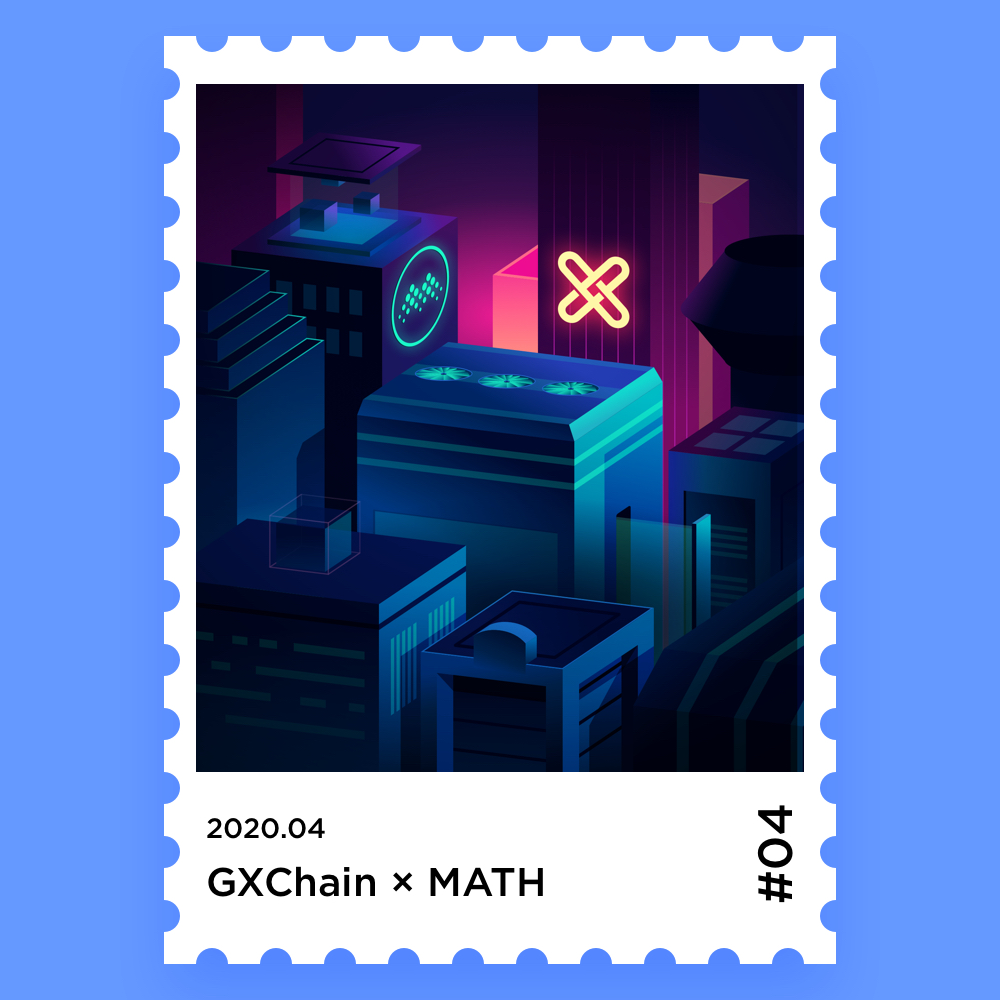005 MATH x DARWINIA

####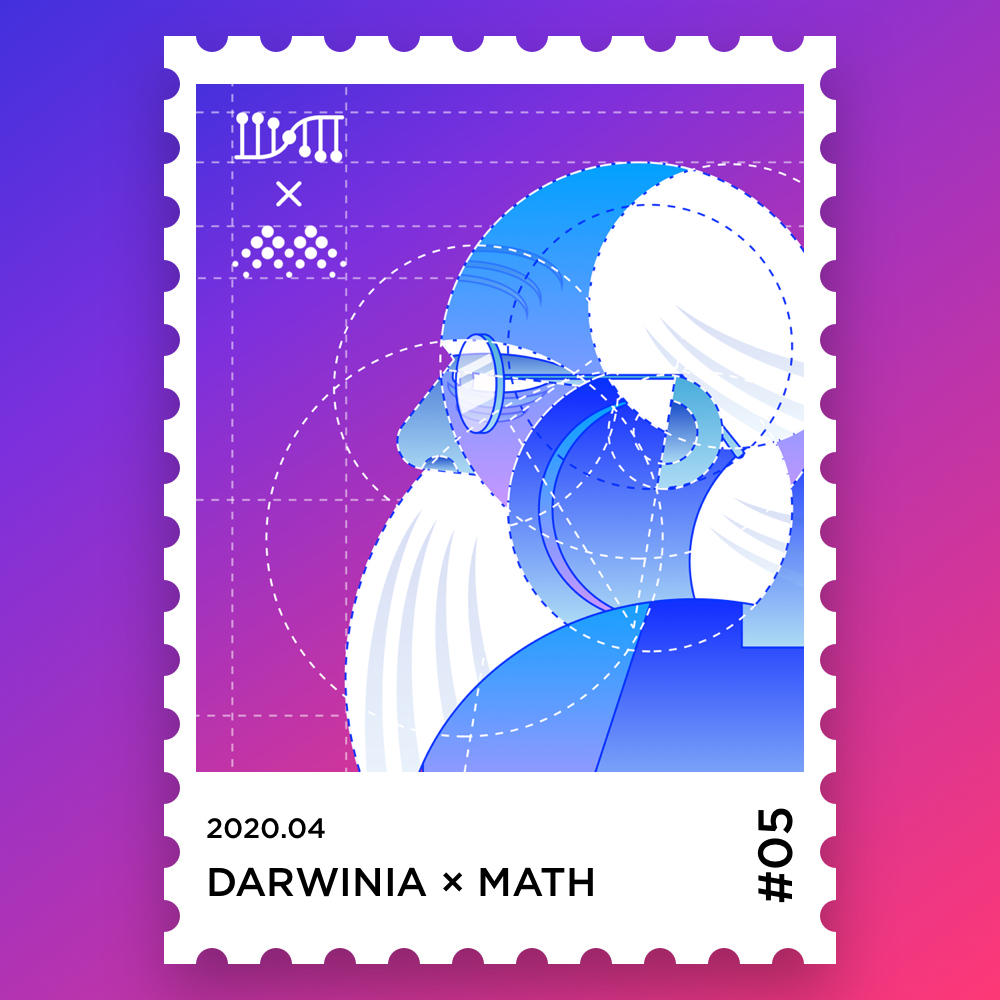006 MATH x STAKEFISH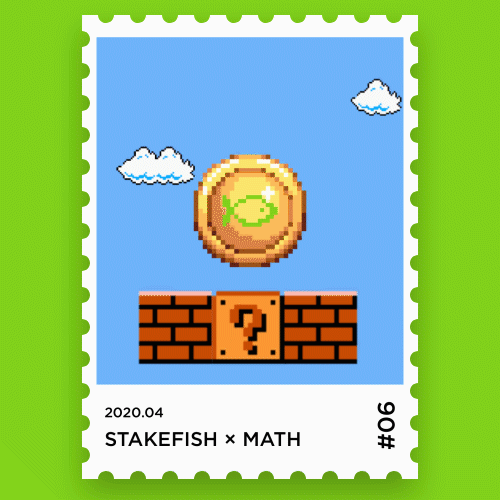007 MATH x CoinBene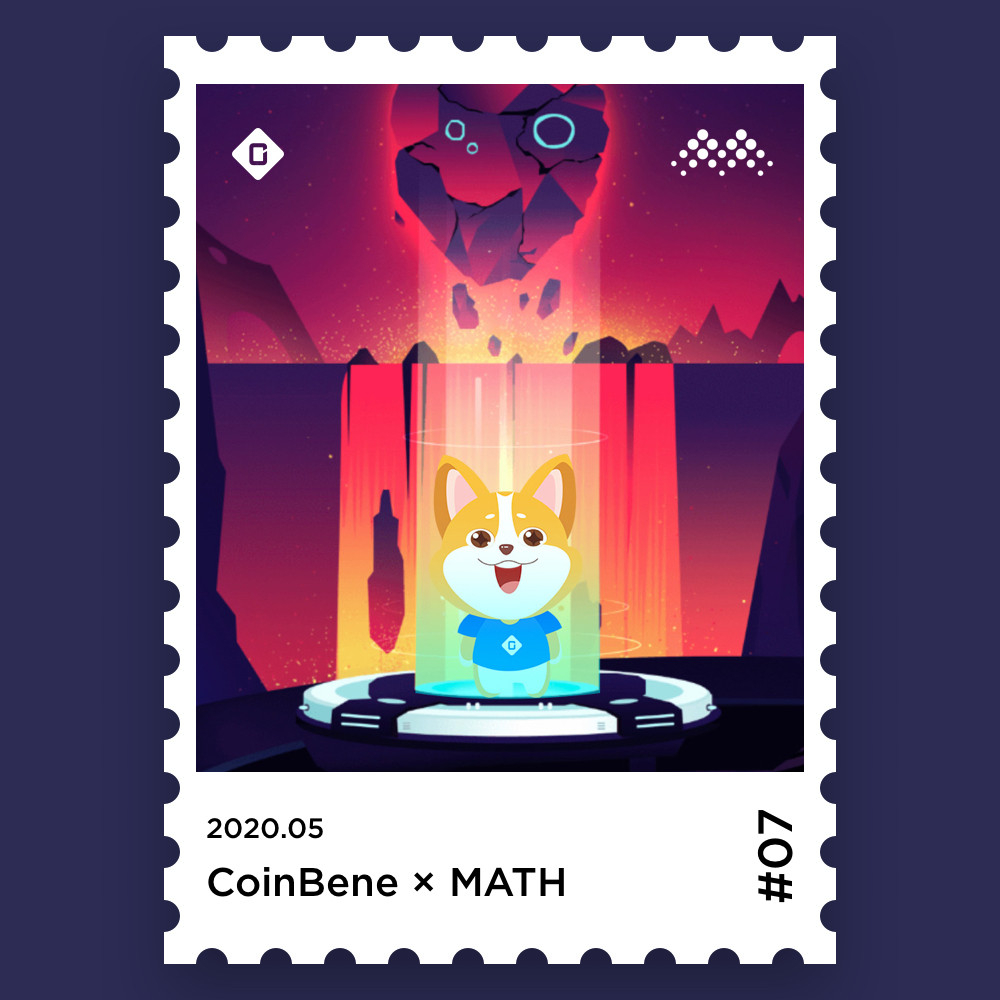008 MATH x IPFSNews009 MATH x ChainX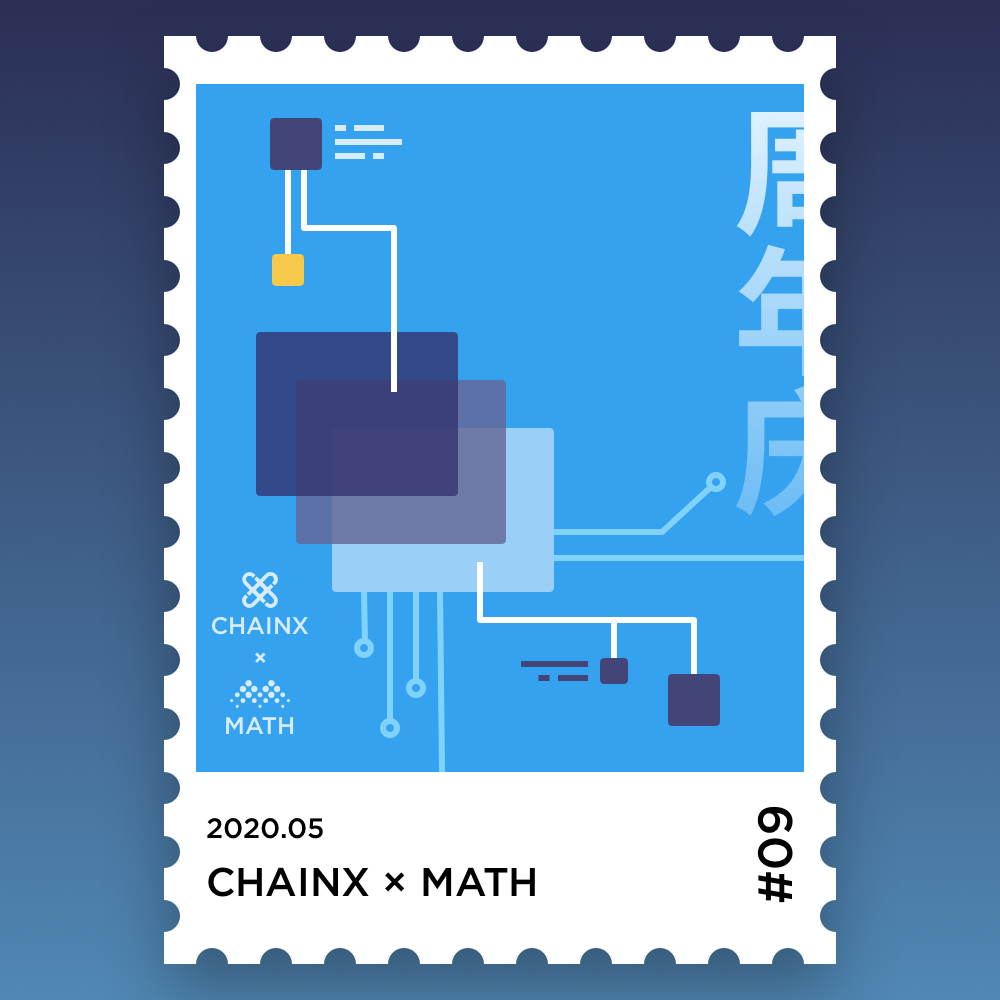010 MATH x BTCC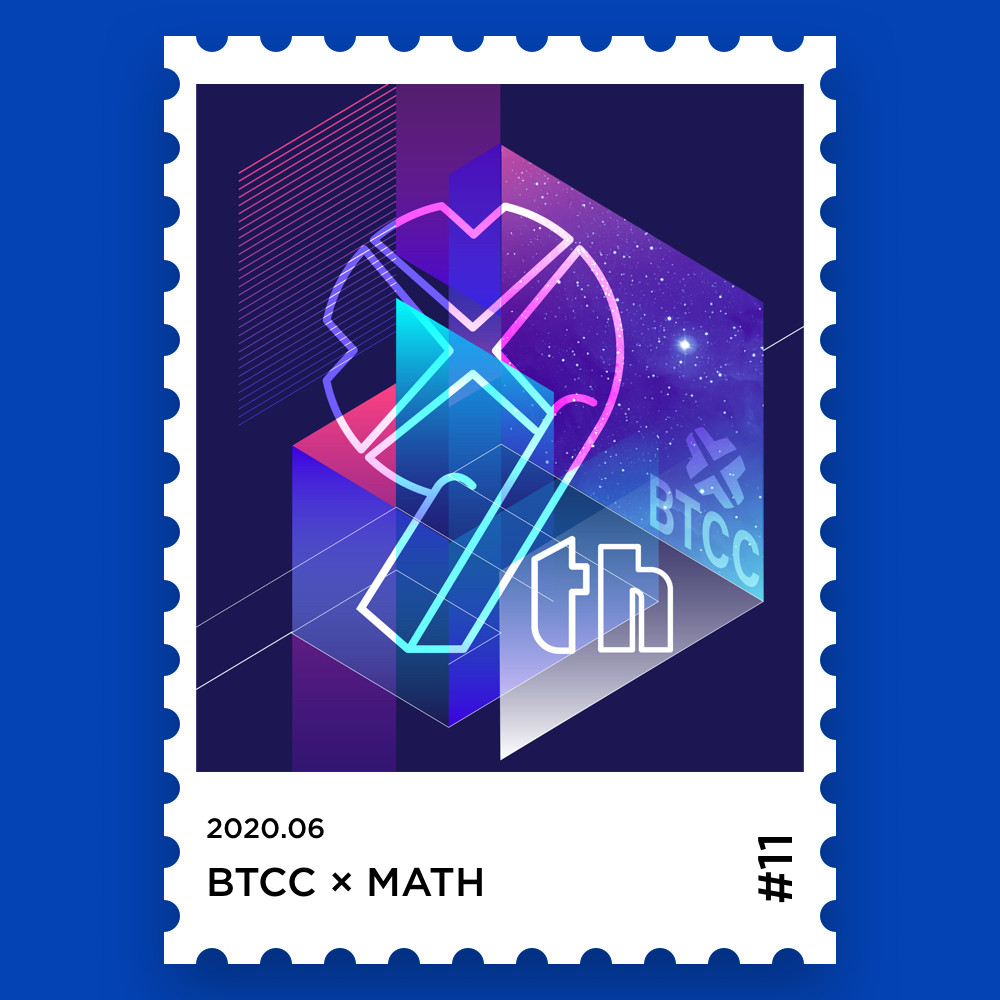011 MATH x AAVE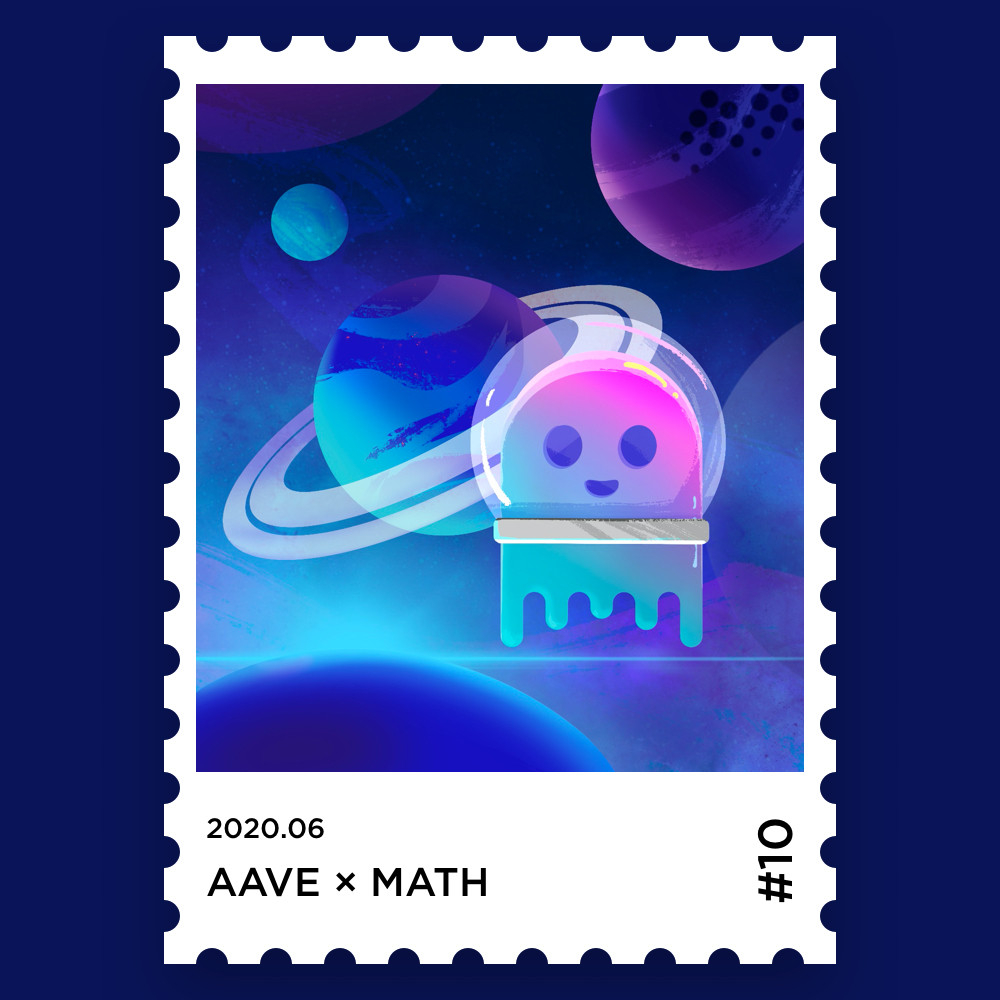012MATH x EOSC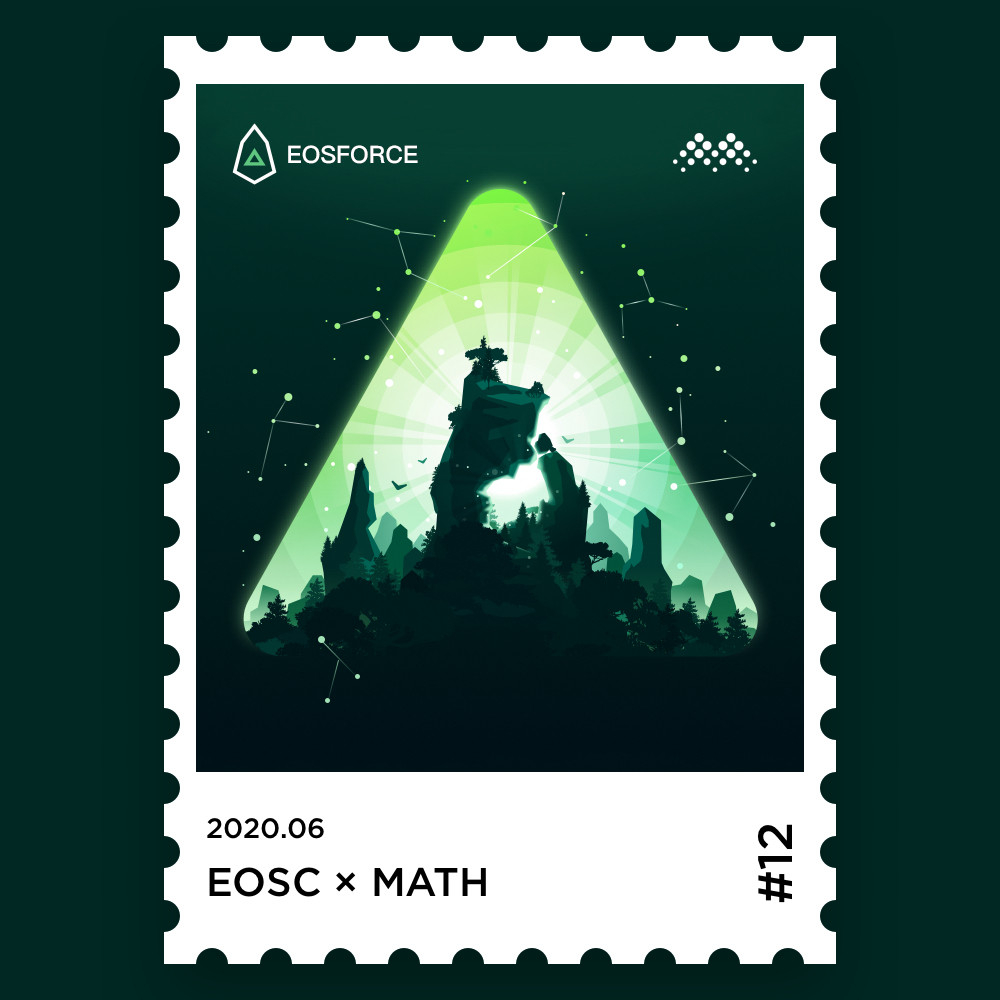013 MATH x HBTC